# How to Create a Perfect Decision Tree

### Decisions are hard. With the right understanding of their formulaic properties, decision trees can be a great help.

· AI Zone · Analysis
Save
22.68K Views

A Decision Tree has many analogies in real life and it has influenced a wide area of Machine Learning, covering both Classification and Regression. In decision analysis, a decision tree can be used to visually and explicitly represent decisions and decision making.

## What Is a Decision Tree?

A decision tree is a map of the possible outcomes of a series of related choices. It allows an individual or organization to weigh possible actions against one another based on their costs, probabilities, and benefits.

As the name goes, it uses a tree-like model of decisions. They can be used either to drive informal discussion or to map out an algorithm that predicts the best choice mathematically.

A decision tree typically starts with a single node, which branches into possible outcomes. Each of those outcomes leads to additional nodes, which branch off into other possibilities. This gives it a tree-like shape.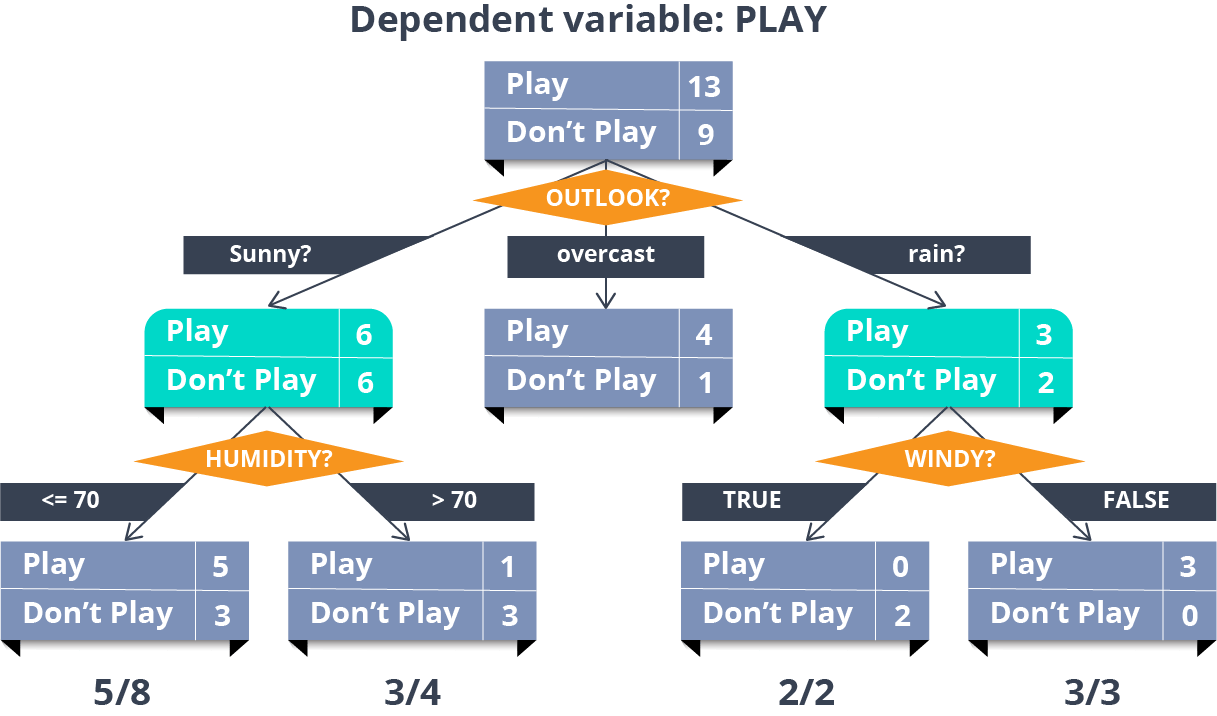There are three different types of nodes: chance nodes, decision nodes, and end nodes. A chance node, represented by a circle, shows the probabilities of certain results. A decision node, represented by a square, shows a decision to be made, and an end node shows the final outcome of a decision path.

## Advantages and Disadvantages of Decision Trees

### Advantages

• Decision trees generate understandable rules.
• Decision trees perform classification without requiring much computation.
• Decision trees are capable of handling both continuous and categorical variables.
• Decision trees provide a clear indication of which fields are most important for prediction or classification.

### Disadvantages

• Decision trees are less appropriate for estimation tasks where the goal is to predict the value of a continuous attribute.
• Decision trees are prone to errors in classification problems with many class and a relatively small number of training examples.
• Decision trees can be computationally expensive to train. The process of growing a decision tree is computationally expensive. At each node, each candidate splitting field must be sorted before its best split can be found. In some algorithms, combinations of fields are used and a search must be made for optimal combining weights. Pruning algorithms can also be expensive since many candidate sub-trees must be formed and compared.

## Creating a Decision Tree

Let us consider a scenario where a new planet is discovered by a group of astronomers, where the question is whether it could be the next Earth. The answer to this question would revolutionize the way people live.

There are n number of deciding factors which need to be thoroughly researched to take an intelligent decision. These factors can be whether water is present on the planet, what the temperature is, whether the surface is prone to continuous storms, whether flora and fauna survives the climate or not, etc.

Let us create a decision tree to find out whether we have discovered a new habitat.

The habitable temperature falls into the range 0 to 100 Celsius.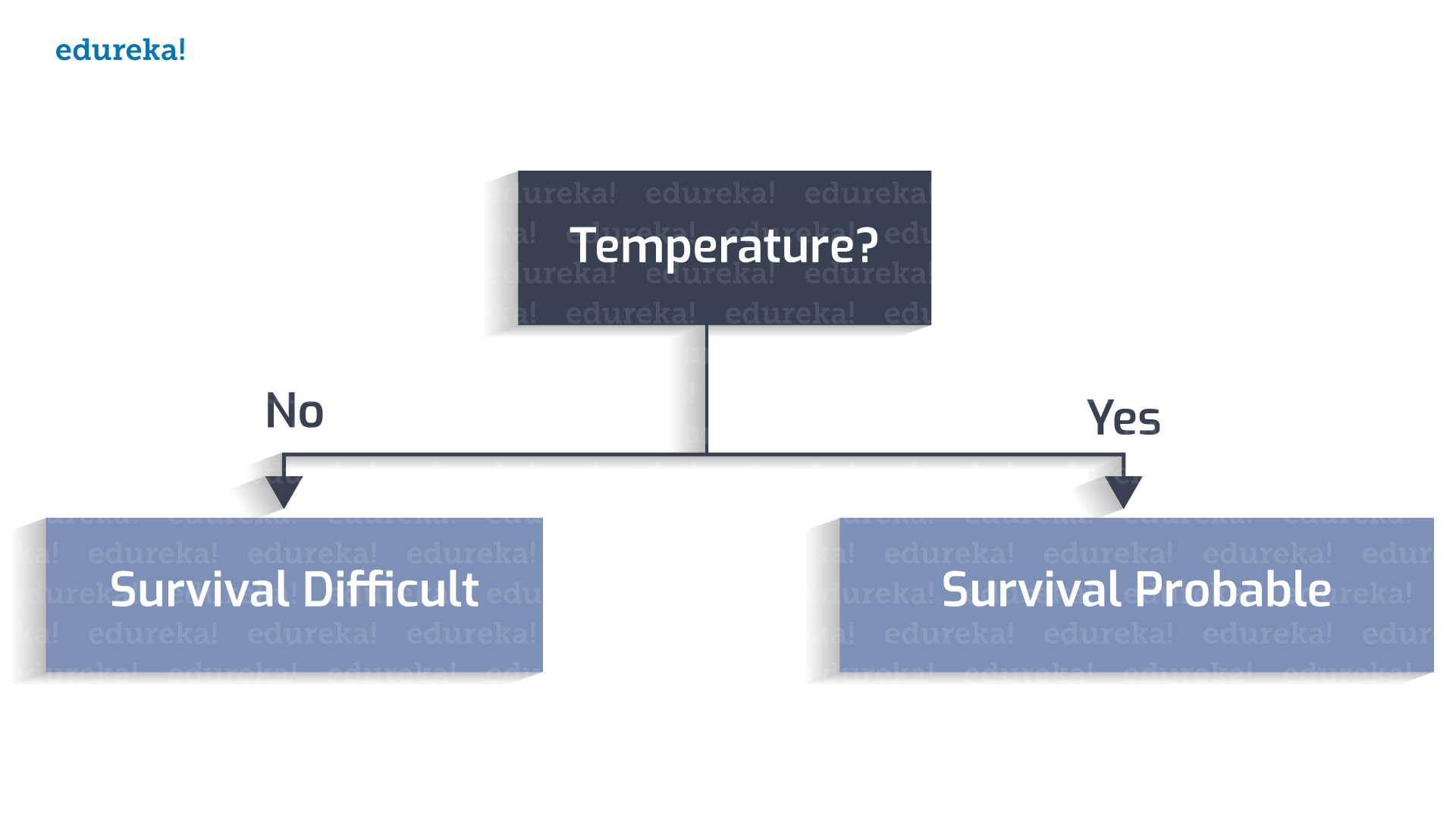Is water present or not?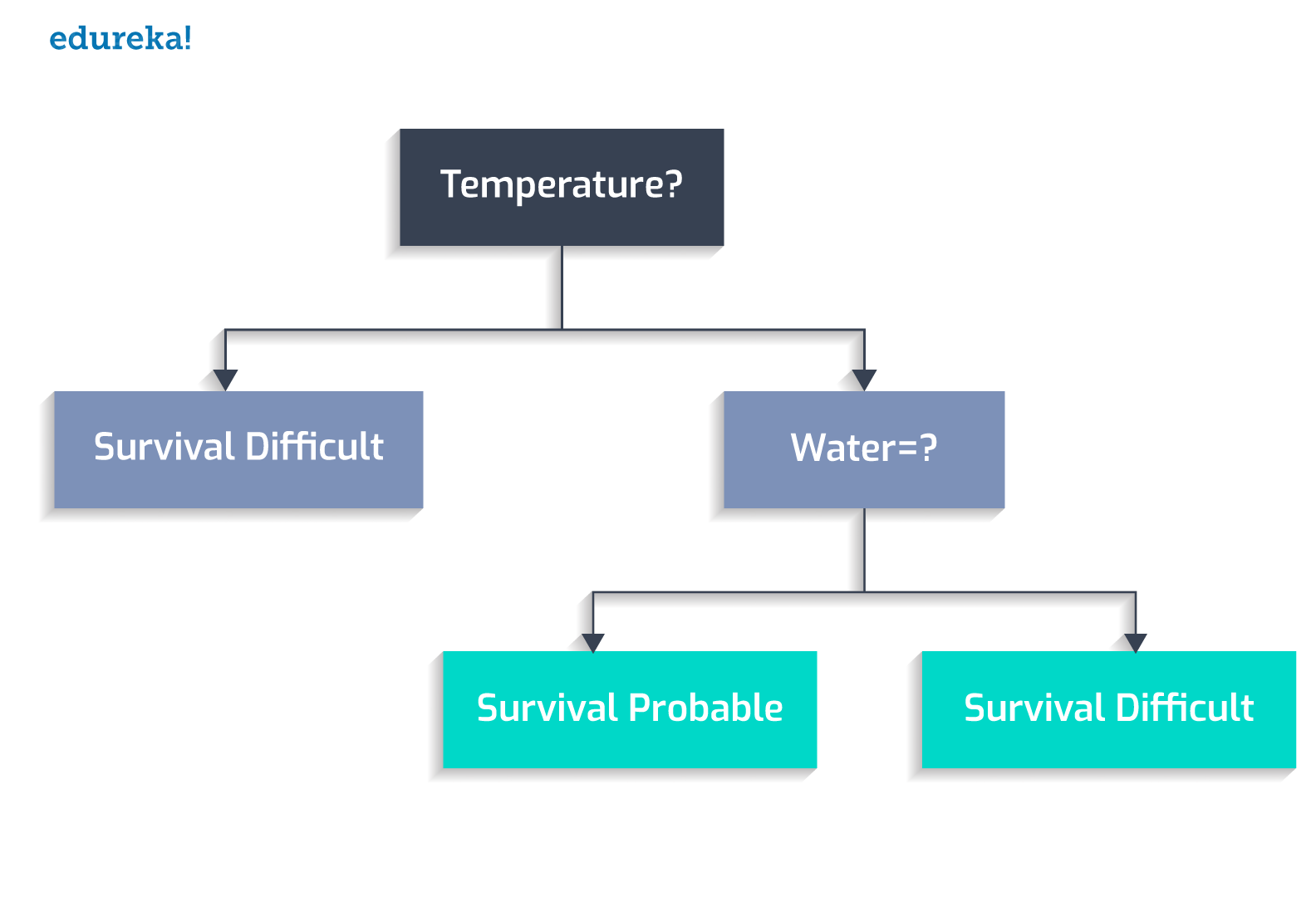Does flora and fauna flourish?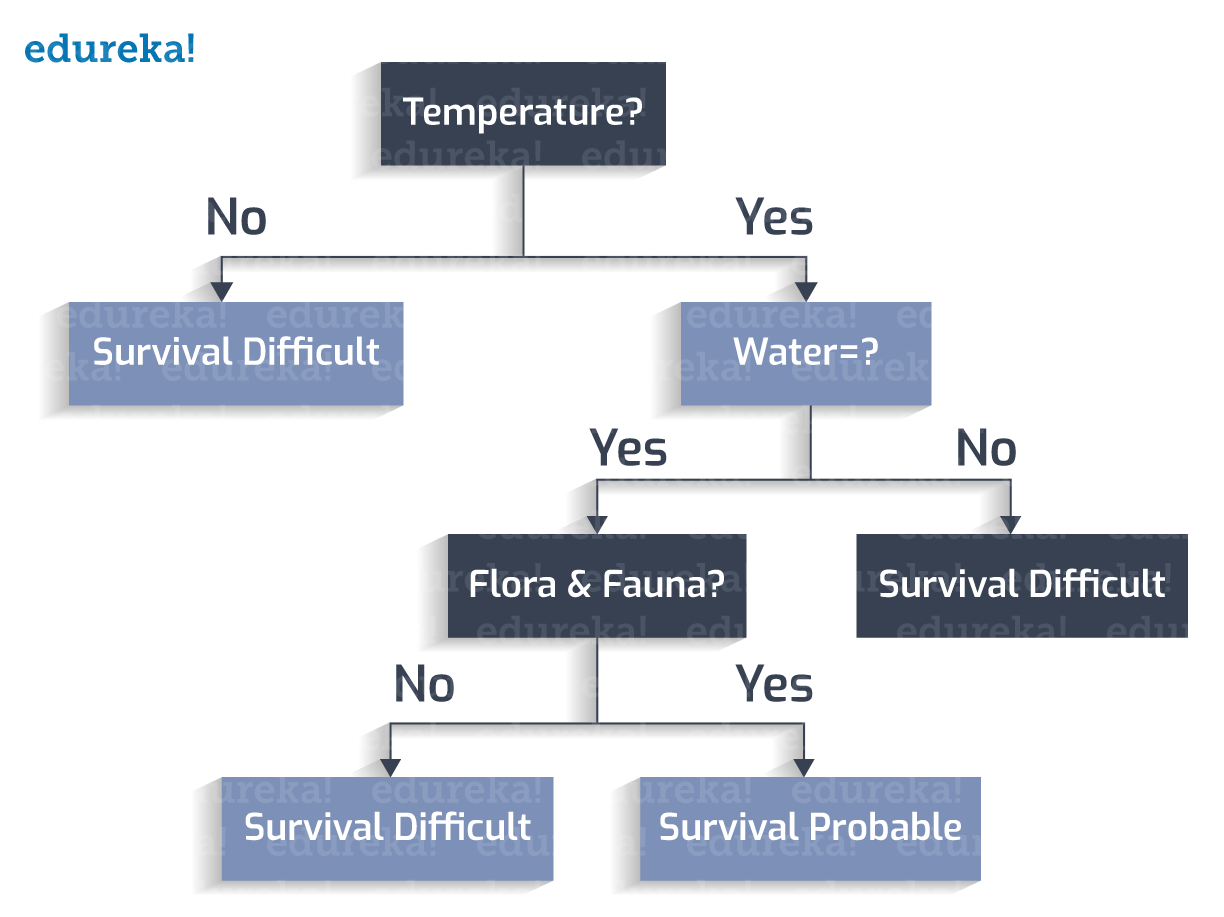Does the planet have a stormy surface?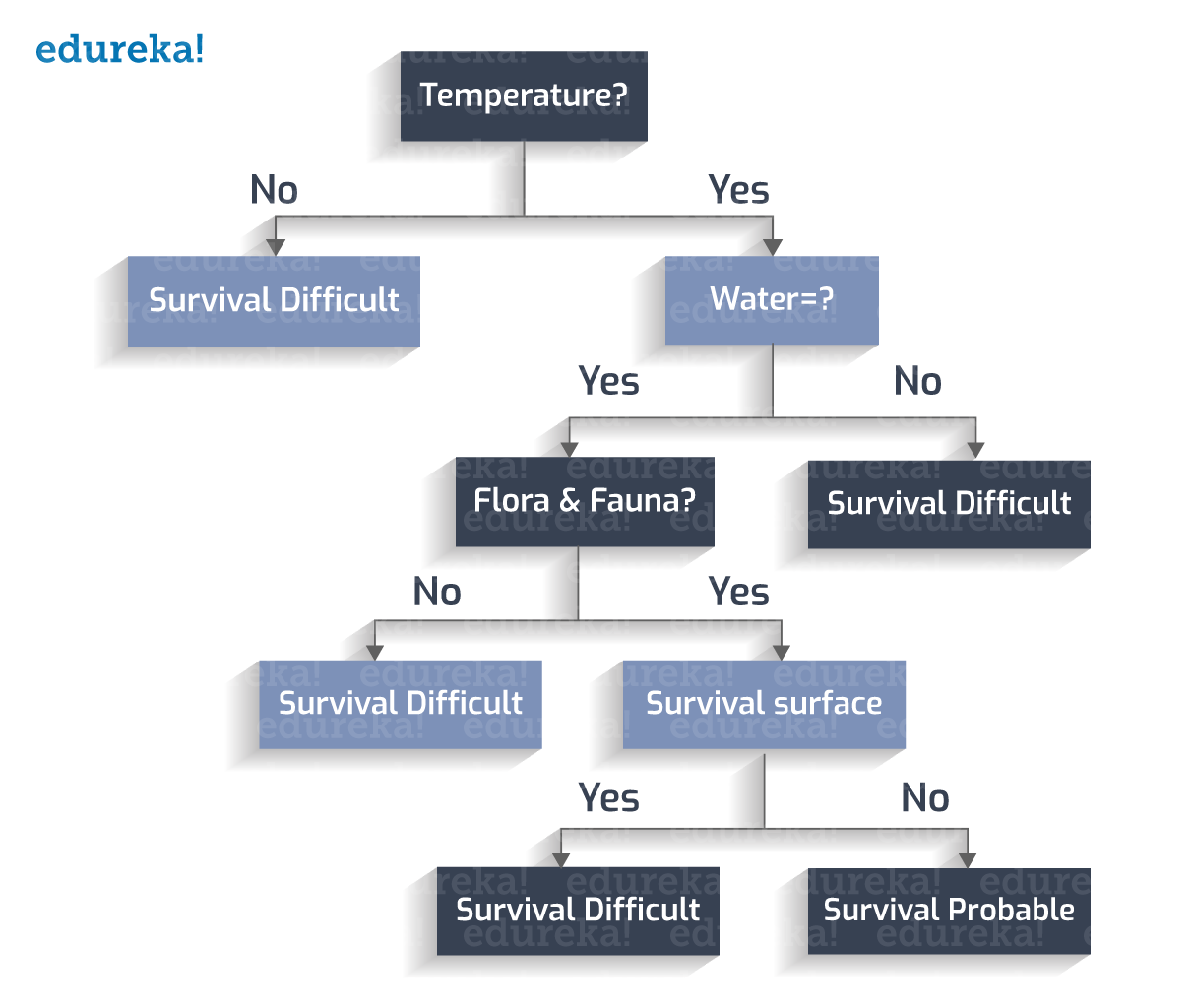Thus, we have a completed decision tree.

## Classification Rules

Classification rules are the cases in which all the scenarios are taken into consideration and a class variable is assigned to each.

### Class Variable

Each leaf node is assigned a class variable. A class variable is the final output which leads to our decision.

Let us derive the classification rules from the Decision Tree created:

1. If Temperature is not between 273 to 373K, -> Survival Difficult

2. If Temperature is between 273 to 373K, and water is not present, -> Survival Difficult

3. If Temperature is between 273 to 373K, water is present, and flora and fauna is not present -> Survival Difficult

4. If Temperature is between 273 to 373K, water is present, flora and fauna is present, and a stormy surface is not present -> Survival Probable

5. If Temperature is between 273 to 373K, water is present, flora and fauna is present, and a stormy surface is present -> Survival Difficult

## Decision Tree

A decision tree has the following constituents:

• Root Node: The factor of "temperature" is considered as the root in this case.
• Internal Node: The nodes with one incoming edge and 2 or more outgoing edges.
• Leaf Node: This is the terminal node with no out-going edge.

As the decision tree is now constructed, starting from the root node, we check the test condition and assign the control to one of the outgoing edges, and so the condition is again tested and a node is assigned. The decision tree is said to be complete when all the test conditions lead to a leaf node. The leaf node contains the class labels, which vote in favor or against the decision.

Now, you might wonder why we started with the "temperature" attribute at the root? If you choose any other attribute, the decision tree constructed will be different.

That's correct. For a particular set of attributes, there can be numerous different trees created. We need to choose the optimal tree which is done by following an algorithmic approach. We will now see "the greedy approach" to creating a perfect decision tree.

## The Greedy Approach

"The Greedy Approach is based on the concept of Heuristic Problem Solving by making an optimal local choice at each node. By making these local optimal choices, we reach the approximate optimal solution globally." — Wikipedia

The algorithm can be summarized as:

1. At each stage (node), pick out the best feature as the test condition.

2. Now split the node into the possible outcomes (internal nodes).

3. Repeat the above steps until all the test conditions have been exhausted into leaf nodes.

When you start to implement the algorithm, the first question is: "How do you pick the starting test condition?"

The answer to this question lies in the values of Entropy and Information Gain. Let us see what are they and how they impact our decision tree creation.

Entropy: Entropy in Decision Tree stands for homogeneity. If the data is completely homogenous, the entropy is 0; otherwise, if the data is divided (50-50%) entropy is 1.

Information Gain: Information Gain is the decrease/increase in Entropy value when the node is split.

An attribute should have the highest information gain to be selected for splitting. Based on the computed values of Entropy and Information Gain, we choose the best attribute at any particular step.

Let us consider the following data: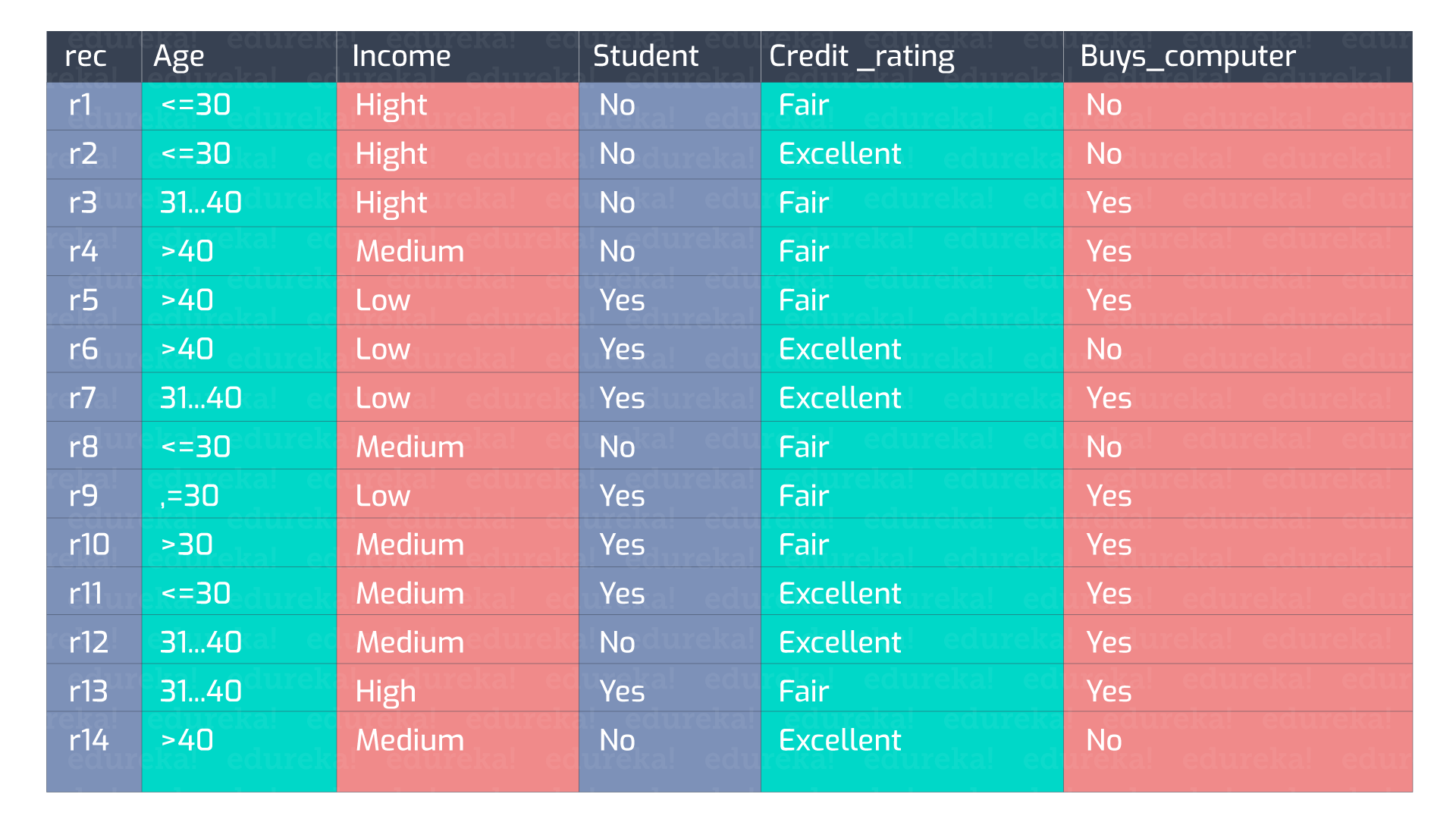There can be n number of decision trees that can be formulated from these set of attributes.

### Tree Creation Trial 1:

Here we take up the attribute "Student" as the initial test condition.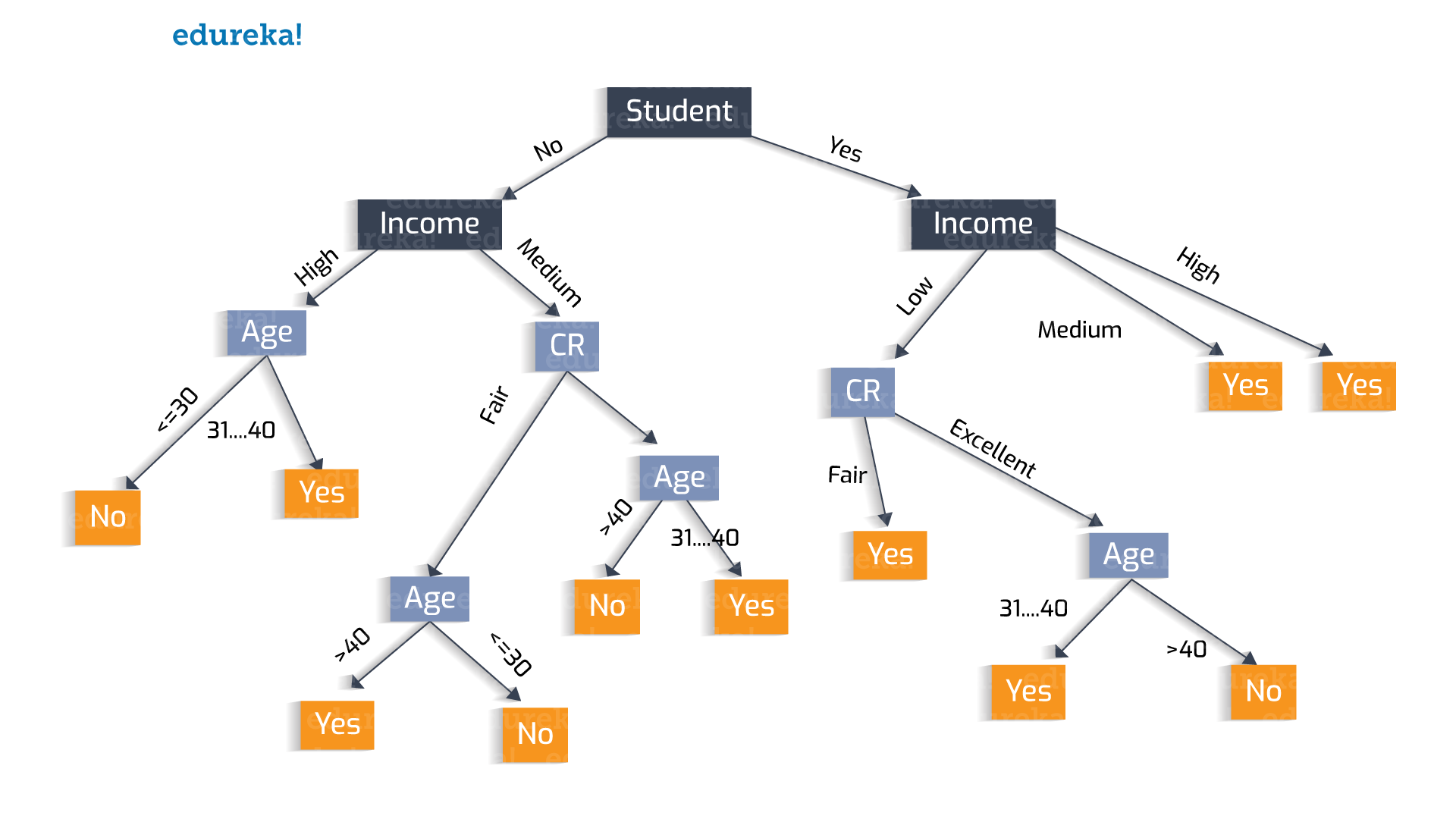### Tree Creation Trial 2 :

Similarly, why to choose "Student"? We can choose "Income" as the test condition.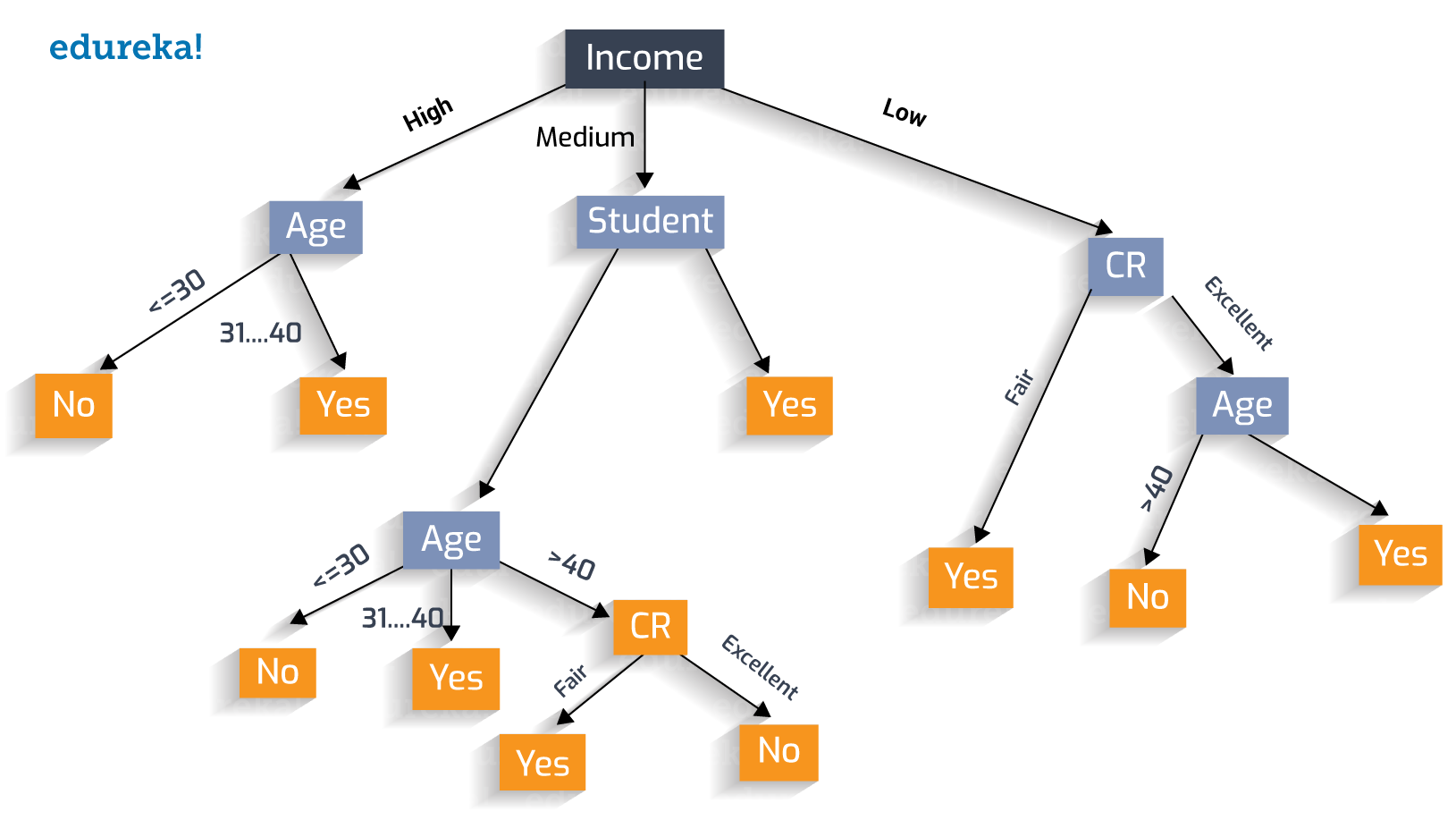## Creating the Perfect Decision Tree With Greedy Approach

Let us follow the Greedy Approach and construct the optimal decision tree.

There are two classes involved: "Yes," saying the person buys a computer, or "No," indicating he does not. To calculate Entropy and Information Gain, we are computing the value of Probability for each of these 2 classes.

»Positive: For " `buys_computer=yes` " probability will come out to be :»Negative: For "`buys_computer=no`" the probability comes out to be:Entropy in D: We now put calculate the Entropy by putting probability values in the formula stated above.We have already classified the values of Entropy, which are:

Entropy = 0: Data is completely homogenous (pure)

Entropy = 1: Data is divided into 50%/50 % (impure)

Our value of Entropy is 0.940, which means our set is almost impure.

Let’s delve deep, to find out the suitable attribute and calculate the Information Gain.

What is information gain if we split on “Age”?

This data represents how many people falling into a specific age bracket buy and do not buy the product.

For example, for people with Age 30 or less, 2 people buy (Yes) and 3 people do not buy (No) the product. The Info (D) is calculated for these 3 categories of people, which is represented in the last column.

The Info (D) for the age attribute is computed by the total of these 3 ranges of age values. Now, the question is what is the "information gain" if we split on "Age" attribute.

The difference of the total Information value (0.940) and the information computed for age attribute (0.694) gives the "information gain."This is the deciding factor for whether we should split at "Age" or any other attribute. Similarly, we calculate the "information gain" for the rest of the attributes:

Information Gain (Age) = 0.246

Information Gain (Income) = 0.029

Information Gain (Student) = 0.151

Information Gain (credit_rating) = 0.048

On comparing these values of gain for all the attributes, we find out that the "information gain" for "Age" is the highest. Thus, splitting at "age" is a good decision.

Similarly, at each split, we compare the information gain to find out whether that attribute should be chosen for split or not.

Thus, the optimal tree created looks like: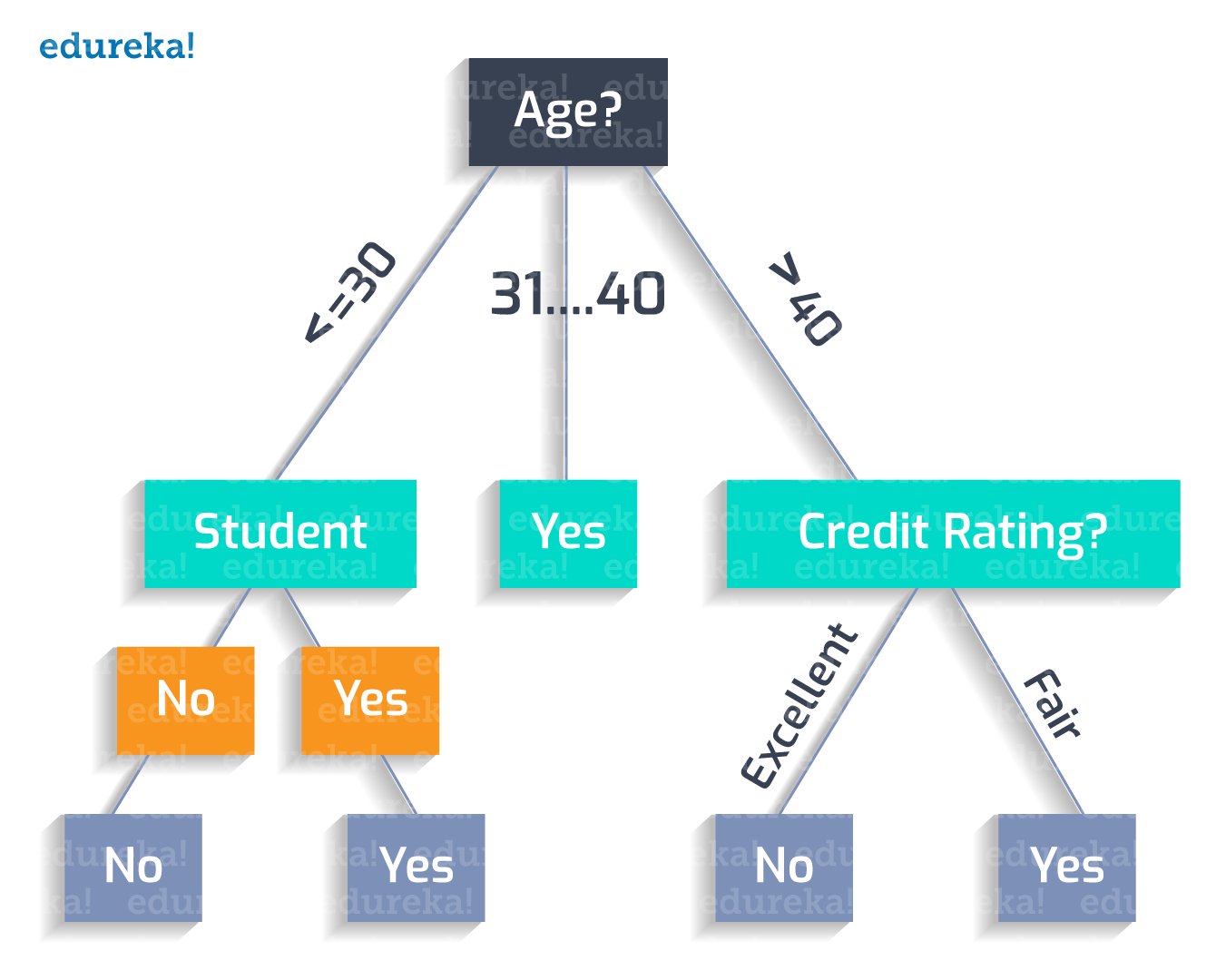The classification rules for this tree can be jotted down as:

If a person’s age is less than 30 and he is not a student, he will not buy the product.

Age (<30) ^ student(no) = NO

If a person’s age is less than 30 and he is a student, he will buy the product.

Age (<30) ^ student(yes) = YES

If a person’s age is between 31 and 40, he is most likely to buy.

Age (31…40) = YES

If a person’s age is greater than 40 and has an excellent credit rating, he will not buy.

Age (>40) ^ credit_rating(excellent) = NO

If a person’s age is greater than 40, with a fair credit rating, he will probably buy.
Age (>40) ^ credit_rating(fair) = Yes

Thus, we achieve the perfect Decision Tree!

Topics:
machine learning, ml algorithm, data science, decision tree

Published at DZone with permission of Upasana Priyadarshiny, DZone MVB.

Opinions expressed by DZone contributors are their own.

Comments